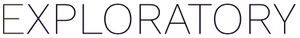# Competing risk survival analysis

#1

Cancer cohort. I am looking to calculate relapse-free survival. I have for each person the following date variables: (a) relapse; (b) death; © last follow-up. STATUS = TRUE if the person has relapsed OR died, FALSE if still alive at last follow-up without any prior relapse. If the person did not relapse or die, columns (a) and (b) will have an NA value for that person. If the person died, columns (b) and © will contain the same date. Questions:

1. How do I find the minimum date of the three date columns, since that is the date I need to use in the competing risk analysis?
2. If I had coded STATUS as 0 = alive and well, 1 = relapsed and 2 = died, how can I run a Kaplan-Meier analysis and plot the curves where 1 is relapsed (event of interest), and the curve is censored at both 0 and 2 (where 2 is the competing risk)? This is how the cmprsk package in R works and many of my data sets are already set up that way.